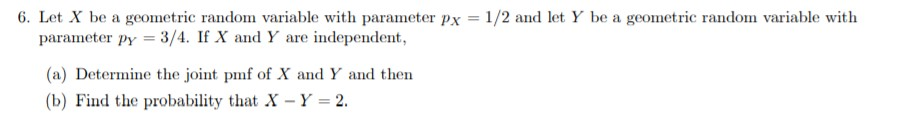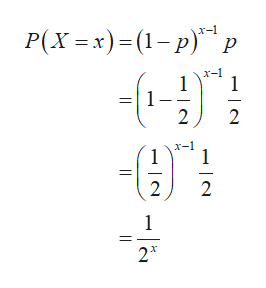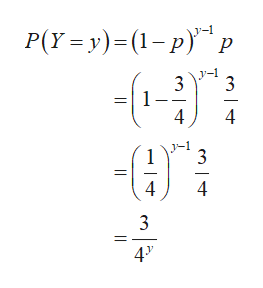# 6. Let X be a geometric random variable with parameter px 1/2 and let Y be a geometric random variable withparameter py 3/4. If X and Y are independent,(a) Determine the joint pmf of X and Y and then(b) Find the probability that X Y 2

Question
14 views

6help_outlineImage Transcriptionclose6. Let X be a geometric random variable with parameter px 1/2 and let Y be a geometric random variable with parameter py 3/4. If X and Y are independent, (a) Determine the joint pmf of X and Y and then (b) Find the probability that X Y 2 fullscreen
check_circle

Step 1

It is given that the variables X and Y are independent.

Step 2

The pmf of X is obtained as follows:help_outlineImage TranscriptioncloseР(х -х)- (1-р)" х-1 р х-1 1 1 2 2 -е х-1 1 1 2 1 2 - l2. fullscreen
Step 3

The pmf of Y is obtained as follows:

...help_outlineImage TranscriptioncloseP(Y y) (1-p) р - 3 1 4 4 1 3 4 4 3 4" fullscreen

### Want to see the full answer?

See Solution

#### Want to see this answer and more?

Solutions are written by subject experts who are available 24/7. Questions are typically answered within 1 hour.*

See Solution
*Response times may vary by subject and question.
Tagged in

### Data